085-1263404

### My Journal

All things Mathematical
23 Oct 2018
##### Question 1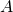and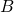are the ends of a uniform bar which is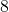inches in length and which weighs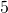lb. The bar is supported by two strings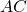and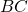attached to a fixed peg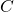. If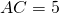inches and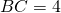inches, find the tensions in the strings.

What weight attached atwould maintain the bar in a horizontal position?

##### Question 2

A non-uniform beam rests with one end in contact with a rough horizontal plane (coefficient of friction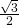) and the other end in contact with a rough vertical wall (coefficient of friction), the inclination of the beam to the horizontal being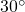. If the beam is just about to slip, in a vertical plane, find the ratio in which the length of the beam is divided by the position of its centre of gravity.

##### Question 3

A car starts from rest and gathers speed. The following table gives the distance travelled by the car from its starting point in the corresponding time :-

Time (in seconds)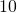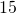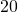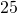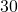Distance (in feet)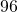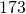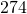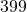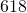Draw a distance-time curve and use it to estimate the velocity of the car at the end of (i)seconds (ii)seconds (iii)seconds.

Plot those three values on a velocity-time curve, and so find approximately the acceleration of the car at the end ofseconds.

##### Question 4

A circular disc of radius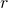is rolling in a straight line on a fixed horizontal plane, the centre of the disc having a uniform velocity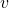. Assuming that no sliding takes place, find the angular velocity of the disc about its centre.

If a point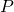is initially at the highest point of the disc, find the linear velocity ofat timein magnitude and direction – giving the inclination to the horizontal.

Show that at any instant there are two points on the rim of the disc whose linear velocities are both equal toin magnitude.

##### Question 5

Starting from rest at the foot of an incline ofin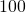, a cyclist travels up the incline with uniform acceleration until he has reached a speed ofm.p.h.; he then continues at a steady speed ofm.p.h., so thatseconds after starting from rest he has travelled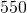feet up the incline. Find his uniform acceleration.

If the cyclist and the cycle together weigh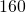lb., and if the frictional resistances to motion are equivalent to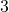lb. wt., find the horse-power at which the cyclist was working when his speed wasm.p.h.

##### Question 6

A mass ofounces is projected vertically upwards with an initial velocity of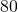ft. per sec. andseconds later a mass ofounce is projected vertically upwards from the same point with an initial velocity of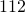ft. per sec. If the masses coalesce on colliding, in how many seconds after the collision will the combined mass return to the point of projection?

If the masses were projected as stated, except that each mass, at its time of projection, is given also a component horizontal velocity, show that the masses would still collide provided that the horizontal velocities were in the same direction and were in the ratio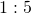.

##### Question 7

A motor cyclist is travelling at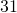m.p.h. round a curve of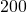feet radius on a track which is inclined to the horizontal at an angle of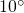(the plane of the curve being horizontal). Find the angle which the cyclist makes with the normal to the track.

If he can travel round the curve at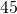m.p.h. without skidding, find the least possible value of the coefficient of friction between the track and the tyres.

##### Question 8

Define Simple Harmonic Motion.

The tension in a spiral spring is directly proportional to the distance through which it is extended beyond its natural length. When a mass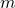is suspended from it, the spring is extended a distance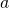beyond its natural length. If the mass is then pulled downwards from its equilibrium position and released, show that its subsequent motion is Simple Harmonic, and find the periodic time in terms of.

##### Question 9

Prove that the pressure is the same at all points at the same depth in a liquid at rest.

A solid cylinder is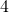inches in height and has a diameter ofinches, and its specific gravity is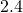. The cylinder is suspended by a vertical string so that it is totally immersed in a liquid of specific gravity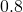. The axis of the cylinder is vertical and the top of the cylinder is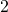inches below the surface of the liquid. Find the total thrust of the liquid (i) on the base of the cylinder, (ii) on the top of the cylinder; and find also the tension in the string.

##### Citation:

State Examinations Commission (2018). State Examination Commission. Accessed at: https://www.examinations.ie/?l=en&mc=au&sc=ru

Malone, D and Murray, H. (2016). Archive of Maths State Exams Papers. Accessed at: http://archive.maths.nuim.ie/staff/dmalone/StateExamPapers/

##### Licence:

“Contains Irish Public Sector Information licensed under a Creative Commons Attribution 4.0 International (CC BY 4.0) licence”.

The EU Directive 2003/98/EC on the re-use of public sector information, its amendment EU Directive 2013/37/EC, its transposed Irish Statutory Instruments S.I. No. 279/2005, S.I No. 103/2008, and S.I. No. 525/2015, and related Circulars issued by the Department of Finance (Circular 32/05), and Department of Public Expenditure and Reform (Circular 16/15 and Circular 12/16).

Note. Circular 12/2016: Licence for Re-Use of Public Sector Information adopts CC-BY as the standard PSI licence, and notes that the open standard licence identified in this Circular supersedes PSI General Licence No: 2005/08/01.